### Home > CCAA > Chapter 7 Unit 6 > Lesson CC2: 7.1.6 > Problem7-73

7-73.

Complete the scaling for each number line below.

1.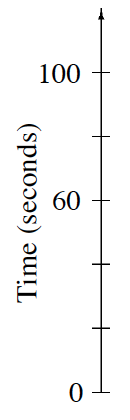Because there are $3$ spaces between $0$ and $60$, each interval will be $60$ divided by $3$. Can you find the interval and complete the scale?

1.Follow the directions in part (a). Make sure to show your work.

Your scale should look like the one filled in below.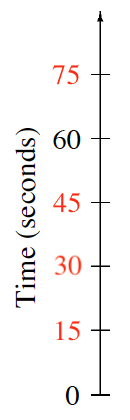1.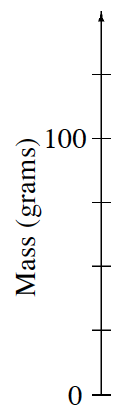Follow the directions given in part (a).

Your scale should look like the one filled in below.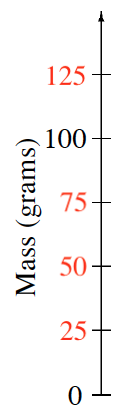1.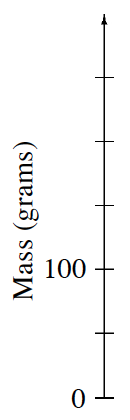Try this one on your own. Refer to parts (a) through (c) for help.